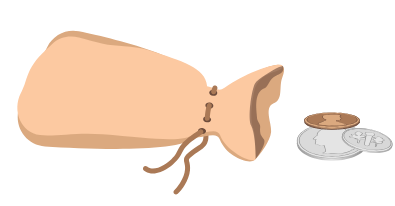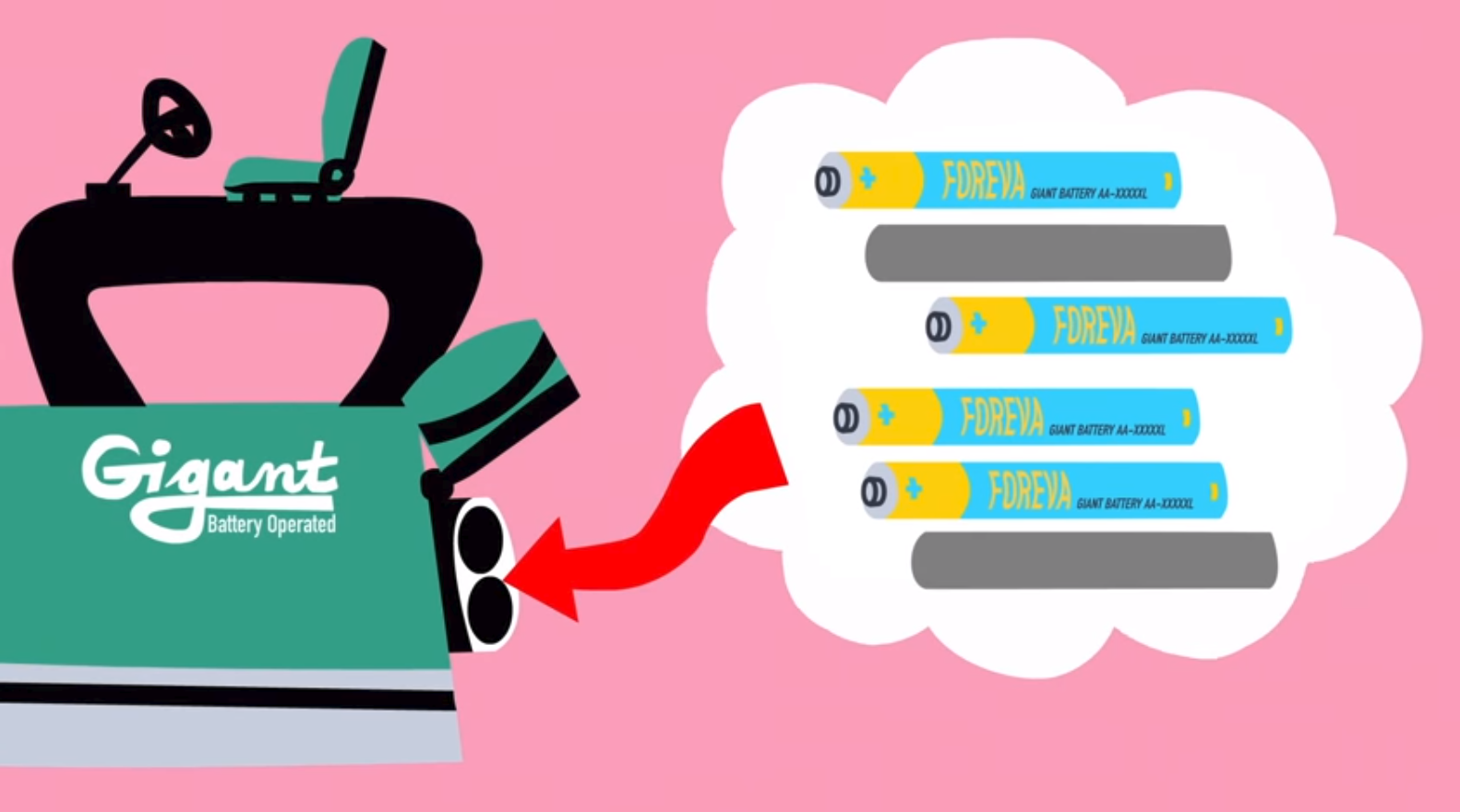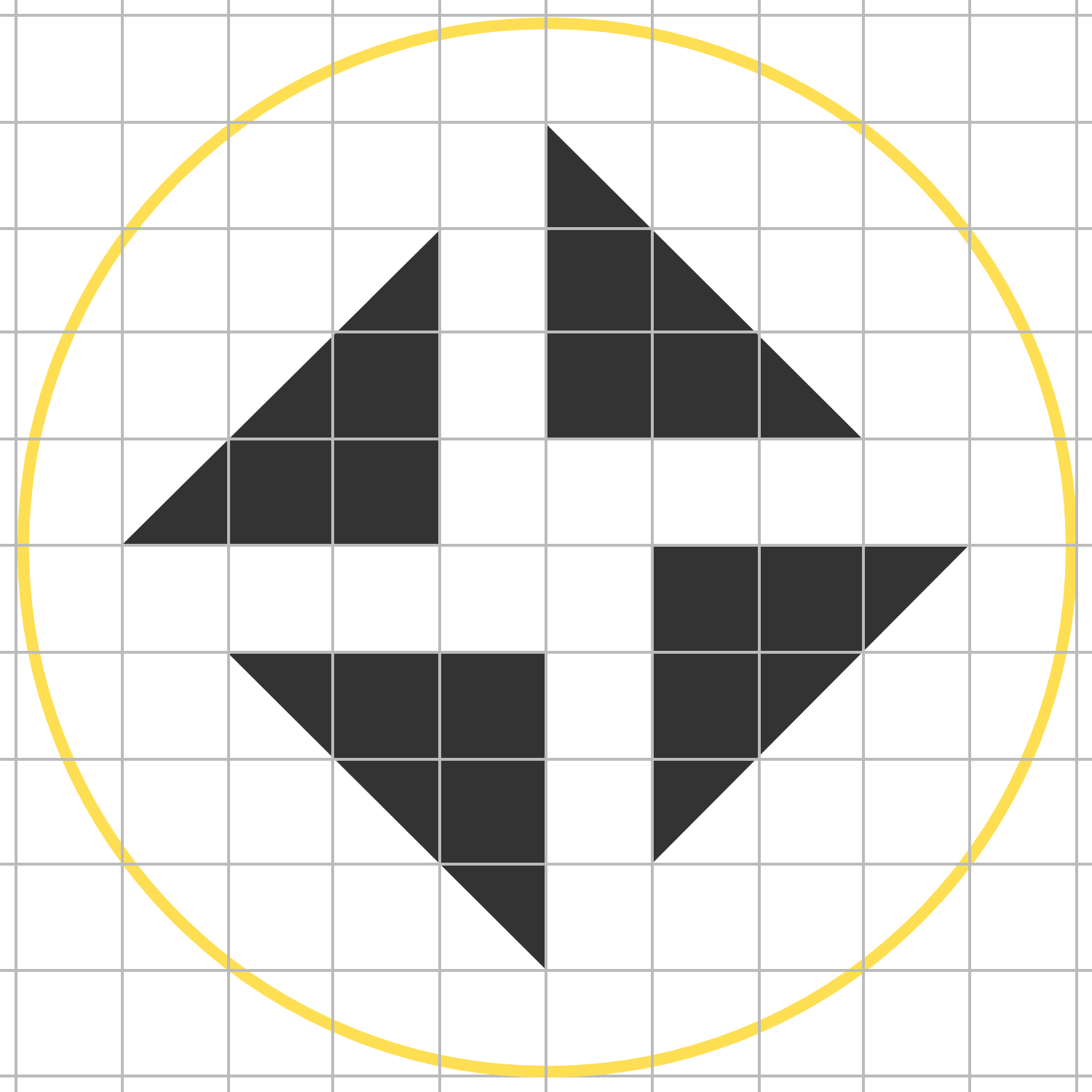# Problems of the Week

Contribute a problem

# 2018-11-26 IntermediateI want to buy a $1.00 newspaper. I have just enough pennies ($0.01), nickels ($0.05), dimes ($0.10), and quarters (\$0.25) to buy the newspaper in every possible exact-change combination of those coins.

How many coins do I have?

Note: "Exact-change combination" doesn't necessarily mean that you must use more than 1 kind of coins listed above. For example, paying 4 quarters is allowed.

You have 8 batteries, and you know 4 are "good" and the other 4 are "bad."

You have a device that takes 6 batteries, but only 4 need to be "good" for it to work. You can test your device by picking 6 of the batteries and trying to turn the device on, but otherwise there is no way of checking if the batteries are good.

In the worst-case scenario, how many tests do you need so you know of a particular set of batteries which will get your device working?The device accepts 6 batteries, although only 4 need to be working. Image via TED-Ed and Artrake Studio.

I am thinking of a 3-digit number $\overline{{\color{#D61F06}A}{\color{#3D99F6}B}{\color{#20A900}C}}.$

I give you the following sum:

$\begin{array}{rcccc} && {\color{#D61F06}A} & {\color{#20A900}C} & {\color{#3D99F6}B} \\ && {\color{#3D99F6}B} & {\color{#D61F06}A} & {\color{#20A900}C} \\ && {\color{#3D99F6}B} & {\color{#20A900}C} & {\color{#D61F06}A} \\ && {\color{#20A900}C} & {\color{#D61F06}A} & {\color{#3D99F6}B} \\ + && {\color{#20A900}C} & {\color{#3D99F6}B} & {\color{#D61F06}A} \\ \hline &1 & 2 & 2 & 3 \end{array}$

What is $\overline{{\color{#D61F06}A}{\color{#3D99F6}B}{\color{#20A900}C}}?$

Note: The digits ${\color{#D61F06}A},$ ${\color{#3D99F6}B},$ and ${\color{#20A900}C}$ are not necessarily distinct.

$p(x) = x^7 + ax^5 + bx^3 + cx$

Given that all of this polynomial's 7 roots are real and three of them are $r = 1,2,$ and $3,$ what is the greatest integer $k$ such that $p(n)$ is divisible by $k$ for all integers $n?$Four isosceles right triangles with legs of length 3 are inside a circle of radius 5 and positioned on a unit grid.

Consider every possible chord of the circle that does not touch a triangle. Then shade every portion of the white inside the circle that is not touched by any of such chords.

The shaded area is $\frac{a}{b},$ where $a$ and $b$ are coprime positive integers.

What is $a+b?$

×

Problem Loading...

Note Loading...

Set Loading...